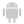WINDEVWEBDEVWINDEV MobileOthersdImageToArray (Function)
In french: dImageVersTableau
Copies the RGB pixels of the image into a one-dimensional or two-dimensional array. The array of colors is cleared then it is filled with the integers representing the colors (24 bits) of the image.
Example
arrLightness is array of * by * int

dImageToArray("c:\test.png", arrLightness, dComponentRed + dGrayScale)

dStartDrawing(IMG_NoName2)
nx, ny are int
FOR nx = 1 _TO_ ArrayInfo(arrLightness, tiNumberRows)
FOR ny = 1 _TO_ ArrayInfo(arrLightness, tiNumberColumns)
dPoint(nx-1, ny-1, arrLightness[nx][ny])
END
END
Syntax

Copying the pixels of an image file into an array

dImageToArray(<Image file> , <Array> [, <Options>])
<Image file>: Character string
Path of image file to use.
<Array>: Array variable
Name of the array of 24-bit color into which the pixels will be copied. This array can be a one-dimensional array or a two-dimensional array. For a two-dimensional array, the dimensions represent the X and Y axes of the image.
<Options>: Optional Integer constant (or combination of constants)
Options for calculating the array:
 dComponentBlue The Blue component of image will be copied into the array. dComponentGreen The Green component of image will be copied into the array. dComponentRed The Red component of image will be copied into the array. dComponentRGB All image components will be copied into the array. dGrayScale The image in grayscale will be copied into the array.

Copying the pixels of an image in memory into an array

dImageToArray(<Image in memory> , <Array> [, <Options>])
<Image in memory>: Buffer or character string
Byte string containing the image to use. This parameter can correspond to an image saved in memory (dSaveImageBMP, dSaveImageGIF, etc.).
<Array>: Array variable
Name of the array of 24-bit color into which the pixels will be copied. This array can be a one-dimensional array or a two-dimensional array. For a two-dimensional array, the dimensions represent the X and Y axes of the image.
<Options>: Optional Integer constant (or combination of constants)
Options for calculating the array:
 dComponentBlue The Blue component of image will be copied into the array. dComponentGreen The Green component of image will be copied into the array. dComponentRed The Red component of image will be copied into the array. dComponentRGB All image components will be copied into the array. dGrayScale The image in grayscale will be copied into the array.
Remarks
• The alpha layer of the image is not returned by this function.
• Change of behavior in version 23: When converting a PDF file (i.e. a vector image) into an array of pixels, a conversion factor must be applied in order to transform a unit of the PDF into a pixel.
From version 23 onwards, the conversion factor has been optimized, which results in a reduction in the size of the image in pixels.
To go back to the behavior of version 22 (non-optimized), you can use the following code (this code uses an Image variable to convert the PDF into the desired dimensions):
lCMyImageh is string = fCurrentDir() + ["\"] + "myfile.pdf"
iiMage is Image = dLoadImage(lCMonImageh, imgConvert, 2479, 3504)
bufImage is Buffer = dSaveImagePNG(iiMage, inMemory)
lSourcePixelArray is array of * by * integers
dImageToArray(bufImage, lSourcePixelArray, dComponentRGB + dGrayScale)
Trace(ArrayInfo(lSourcePixelArray, tiNumberRows) + TAB + ...
ArrayInfo(lSourcePixelArray, tiNumberColumns))
Business / UI classification: Neutral code
Component: wd280pnt.dll
Minimum version required
• Version 16